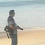Latest Articles

# Specific and General Sutra in Vedic Maths

Info Post
Few days back , we learnt about Only the last term along with some algebraic proof. Continuing with our Vedic Maths, today we will start with another new sutra named as Specific and General Sutra.

Specific and General is the eleventh sutra in Vedic Maths which is also known as Vyastisamastih Sutra. It teaches us how to use the average or exact middle binomial for breaking up the biquadratic down into simple quadratic by the easy device of mutual cancellations of odd powers . In simpler terms, it works with using a specific or average value to solve our problems. A simple use of this sutra in terms of multiplication is explained below.

Vedic Maths are solved by two techniques namely Specific technique and General Technique. The former is effective but can be used for only particular numbers whereas the latter is having a wider scope. In this sutra, we try using the average number since it depicts average is a specific value representing a range of values .

Let us see what happens when we multiply two numbers (Products),

Example 1:
57 x 63 =?

From the above question, we find that 60 can be chosen as an average value for both 63 and 57. And 63 is 6 numbers higher than 57 and for 63, it is 6 less than the number 57. Halving the difference, it is 6/2 = 3
So,
• Step 1 : Square the average value (here it is 60) : 60 x 60 = 3600
• Step 2 : Square the halved difference (here it is 3) : 3 x 3 = 9 ( i.e : 60 - 57 = 3)
• Step 3 : Subtracting the above results got from Step 1 and Step 2, we get 3600 - 9 = 3591
Therefore , 57 x 63 = 3591

Example 2:
95 x 85 = ?

For the above , we have our average value as 90 and it is 10 higher than 85 and 10 less than 95. Note that the differences are equal. Halving, we get 5. And so,
• Step 1: Squaring the average value = 90 x 90 = 8100
• Step 2 :Squaring the  halved difference = 5 x 5= 25
• Step 5: Subtracting the above results got from Step 1 and Step 2,  8100 - 25 = 8075
Therefore , 95 x 85 = 8075

Now, let us take a three digit number and try using the same technique,
Example 3:
115 x 125 =?

For the above , we have our average value as 120 and it is 10 higher than 125 and 10 less than 115. Note that the differences are equal. Half of 10 is 5.  And so,
• Step 1: Squaring the average value = 120 x 120 = 14400
• Step 2 :Squaring the  halved difference = 5 x 5 = 25
• Step 5: Subtracting the above results got from Step 1 and Step 2,  14400 - 25 = 14375
Therefore , 115 x 125 = 14375

In the coming weeks, we shall extend the topic further for the other technique.

Share:

1.Now this techniques are simply amazing...Thanks for sharing such valuable info.. :)

2.Very useful.

3.Great mathematical article , the best of one is three digit number and try using the same technique,Website Design Company

4.This is the application of famous (a+b)2 and (a-b)2 equations. :-)

Thank You for visiting Momscribe.com !